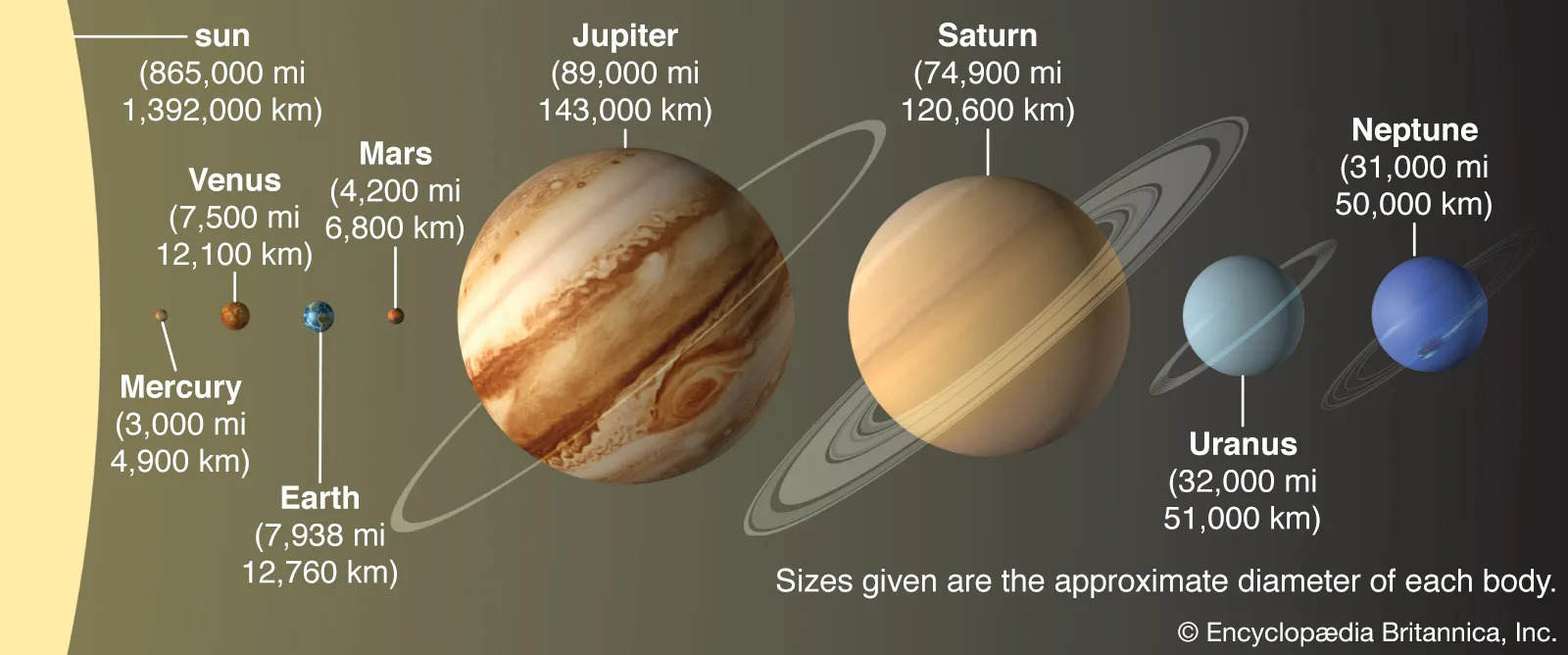2 Marks Questions

Geography
Chapter 1 Class 6 Geography - The Earth and the Solar System

## Name the planets according to their distance from the sun.

The planets according to their distance from the sun are:

• Mercury
• Venus
• Earth
• Mars
• Jupiter
• Saturn
• Uranus
• NeptuneThe distance of a planet from the sun can be measured by its average orbital radius, which is the average distance between the center of the planet and the center of the sun. The orbital radius of a planet can be expressed in kilometers (km) or in astronomical units (AU), where 1 AU is equal to the average distance between Earth and the sun (149.6 million km).

The planets according to their distance from the sun are:

• Mercury - Mercury is the closest planet to the sun. Its average orbital radius is about 57.9 million km, or 0.39 AU.
• Venus - Venus is the second closest planet to the sun. Its average orbital radius is about 108.2 million km, or 0.72 AU.
• Earth - Earth is the third closest planet to the sun. Its average orbital radius is about 149.6 million km, or 1 AU.
• Mars - Mars is the fourth closest planet to the sun. Its average orbital radius is about 227.9 million km, or 1.52 AU.
• Jupiter - Jupiter is the fifth closest planet to the sun. Its average orbital radius is about 778.6 million km, or 5.20 AU.
• Saturn - Saturn is the sixth closest planet to the sun. Its average orbital radius is about 1.43 billion km, or 9.54 AU.
• Uranus - Uranus is the seventh closest planet to the sun. Its average orbital radius is about 2.87 billion km, or 19.19 AU.
• Neptune - Neptune is the eighth and farthest planet from the sun. Its average orbital radius is about 4.50 billion km, or 30.07 AU.

Thus, we can see that Mercury is the closest and Neptune is the farthest planet from the sun.

Learn in your speed, with individual attention - Teachoo Maths 1-on-1 Class